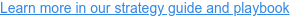# Discover How Much Paper Costs Your Hospital

## (function() { window.addEventListener("message",function(t){ var e="https://www.paypalobjects.com/api/checkout.4.0.141.js"; if(!document.querySelector('script[src="'+e+'"]')&&t.data&&"LOAD_PAYPAL"===t.data.action){ var a=document.createElement("script");a.src=e,a.async=!0,a.dataset={paypalCheckout:!0,noBridge:!0,state:"ppxo_meta",env:"production"},document.body.append(a) }if(t.data&&"CALCONIC_UPDATE_HEIGHT"===t.data.action){ var s=documenet.querySelector('iframe[src="https://app.calconic.com/api/embed/calculator/t.data.payload.id"]'); if(s){s.height=t.data.payload.height}} }); })(); (function() { var qs,j,q,s,d=document, gi=d.getElementById, ce=d.createElement, gt=d.getElementsByTagName, id="calconic_", b="https://cdn.calconic.com/static/js/"; if(!gi.call(d,id)) { j=ce.call(d,"script"); j.id=id; j.type="text/javascript"; j.async=true; j.dataset.calconic=true; j.src=b+"calconic.min.js"; q=gt.call(d,"script"); q.parentNode.insertBefore(j,q) } })(); (function() { var qs,j,q,s,d=document, gi=d.getElementById, ce=d.createElement, gt=d.getElementsByTagName, id="calconic_", b="https://cdn.calconic.com/static/js/"; if(!gi.call(d,id)) { j=ce.call(d,"script"); j.id=id; j.type="text/javascript"; j.async=true; j.dataset.calconic=true; j.src=b+"calconic.min.js"; q=gt.call(d,"script"); q.parentNode.insertBefore(j,q) } })();